# Search Results (64)

View
Selected filters:
• CCSS.Math.Content.6.NS.B.2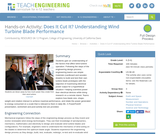Conditions of Use:
Rating

Students gain an understanding of the factors that affect wind turbine operation. Following the steps of the engineering design process, engineering teams use simple materials (cardboard and wooden dowels) to build and test their own turbine blade prototypes with the objective of maximizing electrical power output for a hypothetical situation—helping scientists power their electrical devices while doing research on a remote island. Teams explore how blade size, shape, weight and rotation interact to achieve maximal performance, and relate the power generated to energy consumed on a scale that is relevant to them in daily life. A PowerPoint® presentation, worksheet and post-activity test are provided.

Subject:
Career and Technical Education
Mathematics
Measurement and Data
Numbers and Operations
Physical Science
Material Type:
Activity/Lab
Provider:
TeachEngineering
Author:
Alexander Kon
02/07/2017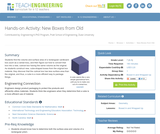Conditions of Use:
Rating

Students find the volume and surface area of a rectangular box (e.g., a cereal box), and then figure out how to convert that box into a new, cubical box having the same volume as the original. As they construct the new, cube-shaped box from the original box material, students discover that the cubical box has less surface area than the original, and thus, a cube is a more efficient way to package things.

Subject:
Engineering
Geometry
Material Type:
Activity/Lab
Lesson Plan
Provider:
TeachEngineering
Provider Set:
TeachEngineering
Author:
Mary R. Hebrank
01/20/2009Conditions of Use:
Remix and Share
Rating

Prior to grade 6, students reasoned about division of whole numbers and decimals to the hundredths in different ways. During this lesson, they revisit two methods for finding quotients of whole numbers without remainder: using base-ten diagrams and using partial quotients. Reviewing these strategies reinforces students’ understanding of the underlying principles of base-ten division—which are based on the structure of place value, the properties of operations, and the relationship between multiplication and division—and paves the way for understanding the long division algorithm. Here, partial quotients are presented as vertical calculations, which also foreshadows long division.This lesson then introduces students to long division. Students see that in long division the meaning of each digit is intimately tied to its place value, and that it is an efficient way to find quotients. In the partial quotients method, all numbers and their meaning are fully and explicitly written out. For example, to find 657÷3 we write that there are at least 3 groups of 200, record a subtraction of 600, and show a difference of 57. In long division, instead of writing out all the digits, we rely on the position of any digit—of the quotient, of the number being subtracted, or of a difference—to convey its meaning, which simplifies the calculation.In addition to making sense of long division and using it to calculate quotients, students also analyze some place-value errors commonly made in long division (MP3).

Subject:
Mathematics
Material Type:
Lesson Plan
Author:
Angela Vanderbloom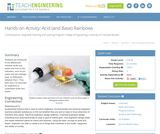Conditions of Use:
Rating

Students are introduced to the differences between acids and bases and how to use indicators, such as pH paper and red cabbage juice, to distinguish between them.

Subject:
Engineering
Material Type:
Activity/Lab
Lesson Plan
Provider:
TeachEngineering
Provider Set:
TeachEngineering
Author:
Amy Kolenbrander
Denise Carlson
Gwendolyn Frank
Janet Yowell
Malinda Schaefer Zarske
Natalie Mach
Sharon Perez
09/26/2008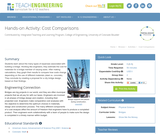Conditions of Use:
Rating

Students learn about the many types of expenses associated with building a bridge. Working like engineers, they estimate the cost for materials for a bridge member of varying sizes. After making calculations, they graph their results to compare how costs change depending on the use of different materials (steel vs. concrete). They conclude by creating a proposal for a city bridge design based on their findings.

Subject:
Architecture and Design
Engineering
Material Type:
Activity/Lab
Provider:
TeachEngineering
Provider Set:
TeachEngineering
Author:
Denali Lander
Denise W. Carlson
Joe Friedrichsen
Jonathan S. Goode
Malinda Schaefer Zarske
Natalie Mach
10/14/2015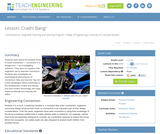Conditions of Use:
Rating

Students learn about the physical force of linear momentum movement in a straight line by investigating collisions. They learn an equation that engineers use to describe momentum. Students also investigate the psychological phenomenon of momentum; they see how the "big mo" of the bandwagon effect contributes to the development of fads and manias, and how modern technology and mass media accelerate and intensify the effect.

Subject:
Engineering
Physics
Material Type:
Activity/Lab
Lesson Plan
Provider:
TeachEngineering
Provider Set:
TeachEngineering
Author:
Ben Heavner
Chris Yakacki
Denise Carlson
Malinda Schaefer Zarske
09/18/2014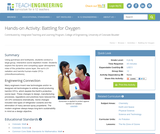Conditions of Use:
Rating

Using gumdrops and toothpicks, students conduct a large-group, interactive ozone depletion model. Students explore the dynamic and competing upper atmospheric roles of the protective ozone layer, the sun's UV radiation and harmful human-made CFCs (chlorofluorocarbons).

Subject:
Engineering
Atmospheric Science
Material Type:
Activity/Lab
Provider:
TeachEngineering
Provider Set:
TeachEngineering
Author:
Amy Kolenbrander
Denise Carlson
Janet Yowell
Malinda Schaefer Zarske
Natalie Mach
Tom Rutkowski
Tyman Stephens
10/14/2015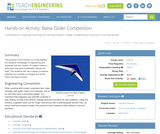Conditions of Use:
Rating

The purpose of this activity is to bring together the students' knowledge of engineering and airplanes and the creation of a glider model to determine how each modification affects the flight. The students will use a design procedure whereby one variable is changed and all the others are kept constant.

Subject:
Engineering
Material Type:
Activity/Lab
Provider:
TeachEngineering
Provider Set:
TeachEngineering
Author:
Alex Conner
Geoffrey Hill
Janet Yowell
Malinda Schaefer Zarske
Tom Rutkowski
10/14/2015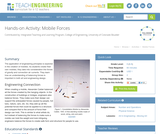Conditions of Use:
Rating

The application of engineering principles is explored in the creation of mobiles. As students create their own mobiles, they take into consideration the forces of gravity and convection air currents. They learn how an understanding of balancing forces is important in both art and engineering design.

Subject:
Engineering
Material Type:
Activity/Lab
Provider:
TeachEngineering
Provider Set:
TeachEngineering
Author:
Denise W. Carlson
Malinda Schaefer Zarske
Natalie Mach
10/14/2015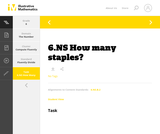Conditions of Use:
No Strings Attached
Rating

This is a task from the Illustrative Mathematics website that is one part of a complete illustration of the standard to which it is aligned. Each task has at least one solution and some commentary that addresses important aspects of the task and its potential use.

Subject:
Numbers and Operations
Material Type:
Activity/Lab
Provider:
Illustrative Mathematics
Provider Set:
Illustrative Mathematics
Author:
Illustrative Mathematics
08/06/2015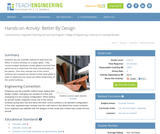Conditions of Use:
Rating

Students use the scientific method to determine the effect of control surfaces on a paper glider. They construct paper airplanes (model gliders) and test their performance to determine the base characteristics of the planes. Then they change one of the control surfaces and compare the results to their base glider in order to determine the cause and effect relationship of the control surfaces.

Subject:
Engineering
Physics
Material Type:
Activity/Lab
Provider:
TeachEngineering
Provider Set:
TeachEngineering
Author:
Alex Conner
Geoffrey Hill
Janet Yowell
Malinda Schaefer Zarske
Tom Rutkowski
10/14/2015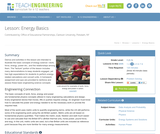Conditions of Use:
Rating

Demos and activities in this lesson are intended to illustrate the basic concepts of energy science -- work, force, energy, power etc. and the relationships among them. The "lecture" portion of the lesson includes many demonstrations to keep students engaged, yet has high expectations for the students to perform energy related calculations and convert units as required. A homework assignment and quiz are used to reinforce and assess these basic engineering science concepts.

Subject:
Engineering
Physics
Material Type:
Activity/Lab
Lesson Plan
Provider:
TeachEngineering
Provider Set:
TeachEngineering
Author:
Jan DeWaters
Susan Powers
09/18/2014Conditions of Use:
Remix and Share
Rating

Subject:
Mathematics
Material Type:
Full Course
Provider:
Pearson
10/06/2016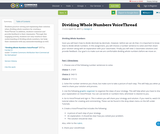Rating

Students practice solving and explaining thier solution when dividing whole numbers by creating a VoiceThread. In addition, students comment and provide feedback to their classmates. Through this engaging activity, students not only reinforce their understanding of dividing whole numbers, but help their classmates with any misunderstandings as well.

Subject:
Mathematics
Material Type:
Activity/Lab
Author:
Carolyn D
04/18/2017Conditions of Use:
Remix and Share
Rating

Fractions and Decimals

Type of Unit: Concept

Prior Knowledge

Students should be able to:

Multiply and divide whole numbers and decimals.
Multiply a fraction by a whole number.
Multiply a fraction by another fraction.
Write fractions in equivalent forms, including converting between improper fractions and mixed numbers.
Understand the meaning and structure of decimal numbers.

Lesson Flow

This unit extends students’ learning from Grade 5 about operations with fractions and decimals.

The first lesson informally introduces the idea of dividing a fraction by a fraction. Students are challenged to figure out how many times a 14-cup measuring cup must be filled to measure the ingredients in a recipe. Students use a variety of methods, including adding 14 repeatedly until the sum is the desired amount, and drawing a model. In Lesson 2, students focus on dividing a fraction by a whole number. They make a model of the fraction—an area model, bar model, number line, or some other model—and then divide the model into whole numbers of groups. Students also work without a model by looking at the inverse relationship between division and multiplication. Students explore methods for dividing a whole number by a fraction in Lesson 3, for dividing a fraction by a unit fraction in Lesson 4, and for dividing a fraction by another fraction in Lesson 6. Students examine several methods and models for solving such problems, and use models to solve similar problems.

Students apply their learning to real-world contexts in Lesson 6 as they solve word problems that require dividing and multiplying mixed numbers. Lesson 7 is a Gallery lesson in which students choose from a number of problems that reinforce their learning from the previous lessons.

Students review the standard long-division algorithm for dividing whole numbers in Lesson 8. They discuss the different ways that an answer to a whole number division problem can be expressed (as a whole number plus a remainder, as a mixed number, or as a decimal). Students then solve a series of real-world problems that require the same whole number division operation, but have different answers because of how the remainder is interpreted.

Students focus on decimal operations in Lessons 9 and 10. In Lesson 9, they review addition, subtraction, multiplication, and division with decimals. They solve decimal problems using mental math, and then work on a card sort activity in which they must match problems with diagram and solution cards. In Lesson 10, students review the algorithms for the four basic decimal operations, and use estimation or other methods to place the decimal points in products and quotients. They solve multistep word problems involving decimal operations.

In Lesson 11, students explore whether multiplication always results in a greater number and whether division always results in a smaller number. They work on a Self Check problem in which they apply what they have learned to a real-world problem. Students consolidate their learning in Lesson 12 by critiquing and improving their work on the Self Check problem from the previous lesson. The unit ends with a second set of Gallery problems that students complete over two lessons.

Subject:
Mathematics
Ratios and Proportions
Material Type:
Unit of Study
Provider:
Pearson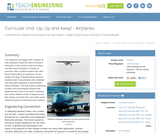Conditions of Use:
Rating

The airplanes unit begins with a lesson on how airplanes create lift, which involves a discussion of air pressure and how wings use Bernoulli's principle to change air pressure. Next, students explore the other three forces acting on airplanes thrust, weight and drag. Following these lessons, students learn how airplanes are controlled and use paper airplanes to demonstrate these principles. The final lessons addresses societal and technological impacts that airplanes have had on our world. Students learn about different kinds of airplanes and then design and build their own balsa wood airplanes based on what they have learned.

Subject:
Engineering
Physics
Material Type:
Full Course
Provider:
TeachEngineering
Provider Set:
TeachEngineering
10/14/2015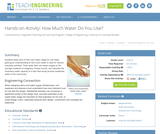Conditions of Use:
Rating

Students keep track of their own water usage for one week, gaining an understanding of how much water is used for various everyday activities. They relate their own water usages to the average residents of imaginary Thirsty County, and calculate the necessary water capacity of a dam that would provide residential water to the community.

Subject:
Engineering
Hydrology
Material Type:
Activity/Lab
Provider:
TeachEngineering
Provider Set:
TeachEngineering
Author:
Denali Lander
Denise W. Carlson
Kristin Field
Megan Podlogar
Sara Born
Tom Rutkowski
09/18/2014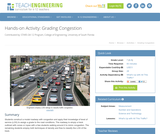Conditions of Use:
Rating

Students construct a model roadway with congestion and apply their knowledge of level of service (LOS) to assign a grade to the road conditions. The roadway is simply a track outlined with cones or ropes with a few students walking around it to mimic congestion. The remaining students employ both techniques of density and flow to classify the LOS of the track.

Subject:
Architecture and Design
Engineering
Material Type:
Activity/Lab
Provider:
TeachEngineering
Provider Set:
TeachEngineering
Author:
Dayna Lee Martinez
Tapas K. Das
09/18/2014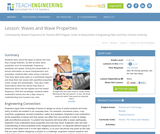Conditions of Use:
Rating

Students learn about the types of waves and how they change direction, as well as basic wave properties such as wavelength, frequency, amplitude and speed. During the presentation of lecture information on wave characteristics and properties, students take notes using a handout. Then they label wave parts on a worksheet diagram and draw their own waves with specified properties (crest, trough and wavelength). They also make observations about the waves they drew to determine which has the highest and the lowest frequency. With this knowledge, students better understand waves and are a step closer to understanding how humans see color.

Subject:
Engineering
Physics
Material Type:
Lesson Plan
Provider:
TeachEngineering
Provider Set:
TeachEngineering
Author:
Courtney Faber
Ellen Zielinski
Marissa H. Forbes
09/18/2014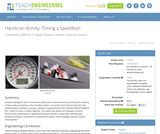Conditions of Use:
Rating

Students strengthen their communicate skills about measurements by learning the meaning of base units and derived units, including speed one of the most common derived units (distance/time). Working in groups, students measure the time for LEGO MINDSTORMS(TM) NXT robots to move a certain distance. The robots are started and stopped via touch sensors and programmed to display the distance traveled. Using their collected data, students complete a worksheet to calculate the robots' (mean/average) speeds at given motor powers.

Subject:
Engineering
Electronic Technology
Material Type:
Activity/Lab
Provider:
TeachEngineering
Provider Set:
TeachEngineering
Author:
James Cox
Sam Sangankar